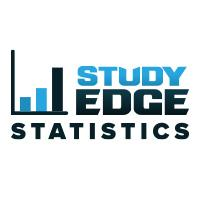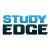7

# Chapter 11: Exploring Bivariate Data

• Subject(s): Math
• Grade Range: 9 - 12
• Release date: 07-13-2017
• Tags: scatterplot, regression, linear, bivariate data, r, linear regression, correlation coefficient, y-intercept, slope, line of best fit, least-squares regression, prediction, outliers, median-median, absolute value, influential points

## Description

In this chapter, students explore the relationship between two quantitative variables. Students will analyze scatterplots for strength, direction, and form; interpret the correlation coefficient; determine the line of best fit using least-squares regression; use the line of best fit to make predictions for a value of y given a value of x; interpret the slope and the y-intercept; learn about alternative methods of finding the line of best fit, including the median-median line and the absolute value line; and identify outliers and influential points and their effects on the regression line and correlation coefficient.

## Standards

M.STAT.7.A, M.STAT.7.B, M.STAT.7.C, M.STAT.7.F, M.STAT.7.D, M.STAT.7.E

## Resources